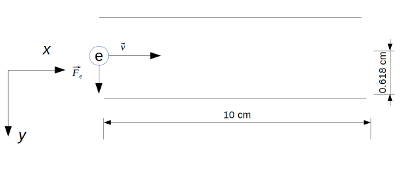# An electron is moving horizontally enters a region of constant electric field field produced by a...

## Question:

An electron is moving horizontally enters a region of constant electric field field produced by a parallel plate capacitor with a positive plate on the bottom and the negative plate on the top. The electric field is directed perfectly vertically. The electron enters the field with a speed of {eq}4.55 \times 10^6\ m/s {/eq} and exits the field {eq}0.618\ cm {/eq} lower than it entered.

a) What is the magnitude of the electric field produced by the capacitor?

b) What is the velocity of the electron when it exits the capacitor's field?

## Electron in a Uniform Electric Field

The electron enters the region of a uniform electric field moving perpendicular to the direction of the field. The motion described by the electron inside the capacitor is like projectile motion, we can analyze it by dividing it between the horizontal motion, along the {eq}x {/eq} axis and a perpendicular motion along the {eq}y {/eq} axis. The electric force is along the {eq}y {/eq} axis, hence, in the horizontal direction the electron is moving with constant velocity, and in the vertical direction the electron moves with constant acceleration under the action of the constant electric field.

## Answer and Explanation: 1

Become a Study.com member to unlock this answer! Create your account

The situation is sketched in the figure below.The electron initial velocity is only in the positive {eq}x {/eq} direction,

{eq}v_{0x}=4.55\ti...

See full answer below.

#### Learn more about this topic:Electric Field & the Movement of Charge

from

Chapter 8 / Lesson 3
14K

After watching this video, you will be able to explain what an electric field is, draw electric field lines and vectors for charged particles, and complete calculations involving electric fields. A short quiz will follow.